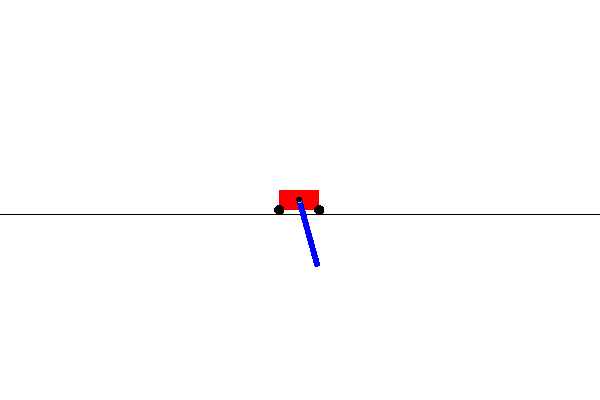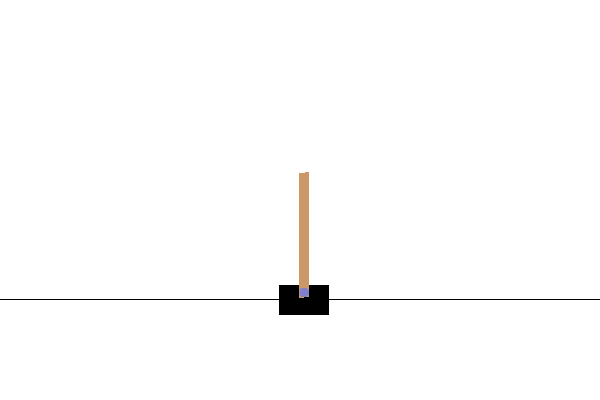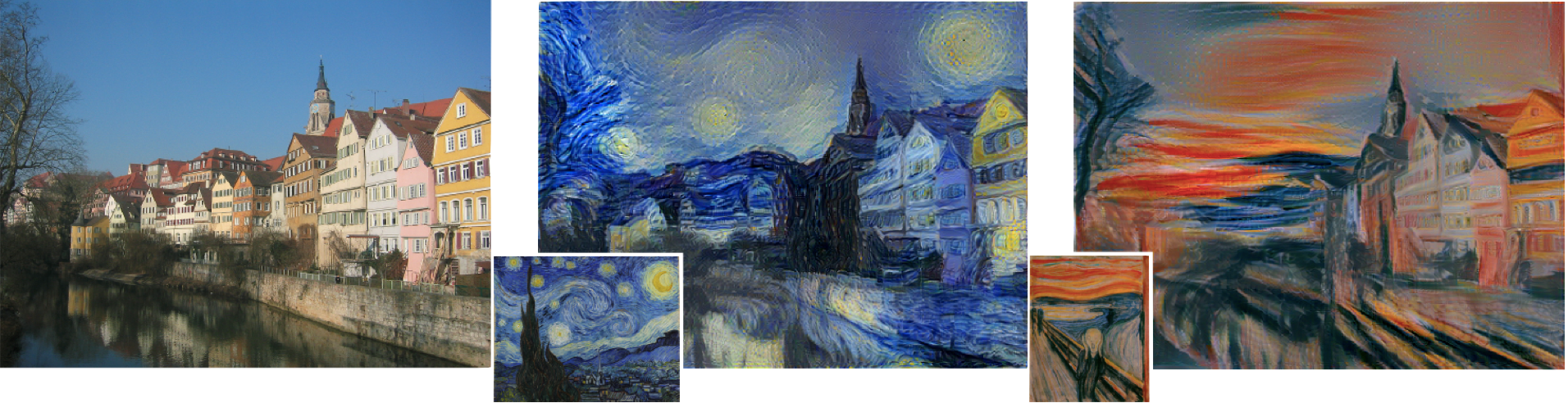## Beyond Vanilla Policy Gradients: Natural Policy Gradients, Trust Region Policy Optimization (TRPO) and Proximal Policy Optimization (PPO)

The point of TRPO is to try to find the largest step size possible that can improve the policy, and it does this by adding a constraint on the KL divergence ...An actor-critic algorithm is a policy gradient algorithm that uses function estimation in place of empirical returns $G_t$ in the policy gradient update.## Policy Gradient Theorem and REINFORCE

In order to use continuous action spaces and have stochastic policies, we have to model the policy $\pi$ directly. We can parametrize our policy using some p...## Deep Q-Learning with Neural Networks

The original formulation of $Q$-learning requires that both the state and action space be small and discrete. However, we run into problems when the action s...## OpenAI Gym and Q-Learning

In the general reinforcement learning paradigm, the agent only has access to the state, the corresponding reward, and the ability to choose an action.## The Mathematical Foundations of Reinforcement Learning

Every action of a rational agent can be thought of as seeking to maximize some cumulative scalar reward signal.## Neural Style Transfer with PyTorch and torchvision

Neural style transfer is a technique for doing style transfer, where we combine the content of one image with the style of another image.## Variational AutoEncoders (VAE) with PyTorch